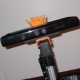ROS Resources: Documentation | Support | Discussion Forum | Index | Service Status | ros @ Robotics Stack Exchange

# rviz odometry is doubling angle?

Hello all, I'm having some problems visualizing odometry with rviz. The problem I am encountering is that rviz appears to be doubling the angle given by the odometry message published:

When the orientation.z of the odometry message is 0.00 (as viewed by rostopic echo odom), the arrow in rviz is aligned vertically with a gridline, as expected

When the orientation.z of the odometry message is 1.58, the arrow in rviz is anti-parallel to the 0.00 arrow from before (~180 degree rotation = ~3.14 radians is approx. 2 * 1.58 radians)

When the arrow on rviz is perpendicular to the initial 0.00 arrow, the orientation.z reads about .707 radians = ~40 degrees.

I'm not too sure if this is a configuration error or not - I originally thought I had written my odometry publisher incorrectly until I started echoing the topic.

Any help would be greatly appreciated.

Relevant Code:

                //since all odometry is 6DOF we'll need a quaternion created from yaw
geometry_msgs::Quaternion odom_quat = tf::createQuaternionMsgFromYaw(
bot->m_odometry_yaw);

//first, we'll publish the transform over tf
geometry_msgs::TransformStamped odom_trans;

odom_trans.transform.translation.x = bot->m_odometry_x;
odom_trans.transform.translation.y = bot->m_odometry_y;
odom_trans.transform.translation.z = 0.0;
odom_trans.transform.rotation = odom_quat;

//send the transform

//next, we'll publish the odometry message over ROS
nav_msgs::Odometry odom;

//set the position
odom.pose.pose.position.x = bot->m_odometry_x;
odom.pose.pose.position.y = bot->m_odometry_y;
odom.pose.pose.position.z = 0.0;
odom.pose.pose.orientation = odom_quat;

//set the velocity
odom.twist.twist.linear.x = bot->m_velocity_x;
odom.twist.twist.linear.y = bot->m_velocity_y;
odom.twist.twist.angular.z = bot->m_velocity_yaw;

//publish the message
odom_pub.publish(odom);


edit retag close merge delete

Sort by » oldest newest most votedI think that you may be confusing your datatypes.

RVIZ takes in a pose with (x,y,z) position and (x,y,z,w) orientation. The orientation is represented by a quaternion. For a quick refresher on quaternions, check out the Wikipedia page.

Probably what is happening is that you are incorrectly publishing your quaternions.

If you have a given set of rotations, in roll/pitch/yaw, you can convert these to a quaternion using the tf::transformations::quaternion_from_euler function. By default it takes the euler angles in sxyz format (roll, pitch, then yaw).

For a bit of example code (in Python):

quat = tf.transformations.quaternion_from_euler(0,0,theta)
### Insert math into Odom msg so it can be published
odom_msg = Odometry()
odom_msg.pose.pose.orientation.x = quat
odom_msg.pose.pose.orientation.y = quat
odom_msg.pose.pose.orientation.z = quat
odom_msg.pose.pose.orientation.w = quat


This is for a differential drive robot, where there is no roll and pitch, and the kinematics are calculated shortly before this snippet of code.

So as an example:

 >>> tf.transformations.quaternion_from_euler(0,0,0)
array([ 0.,  0.,  0.,  1.])
>>> tf.transformations.quaternion_from_euler(0,0,math.pi/2)
array([ 0.        ,  0.        ,  0.70710678,  0.70710678])
>>> tf.transformations.quaternion_from_euler(0,0,2*math.pi)
array([ -0.00000000e+00,   0.00000000e+00,   1.22460635e-16, -1.00000000e+00])


So, just keep in mind that all parts of the quaternion (x,y,z,w) have to be filled out, not just orientation.z.

more

I added the relevant code (C++) to the question - am I making a mistake?Your code looks fine. The problem here is your interpretation of the "orientation" -- which is in fact a quaternion, not euler angles. Therefore, orientation.z is not the rotation about the z axis, but rather a component of the quaternion. For instance, using the tf.transformations module in python, we can see that when your marker is perpendicular (euler rotation about z of 1.57 radians), we have:

quaternion_from_euler(0,0,1.57) = array([ 0. , 0. , 0.70682518, 0.70738827])

Quaternion components are labeled (x,y,z,w), so the quaternion's "z" component will be 0.707 as you saw.

If you run the following (while your node is publishing tf messages):

You can see the transformation in both euler angle and quaternion form.

more

Thanks! That makes sense - I must have my odometry calibrated wrong then (short of rederiving the kinematics, I guess I'll just use a fudge factor of 2.0). I've never really worked with quaternions before, so I was confused.

tf::createQuaternionMsgFromYaw is an method in C++. tf.transformations.quaternion_from_euler is an method in Python. I don't think there is any difference except program language in this program.They are the some function which translate yaw(in euler) to quaternion in this program. I believe that would be something wrong in your computing formula of yaw. In your code: geometry_msgs::Quaternion odom_quat = tf::createQuaternionMsgFromYaw( bot->m_odometry_yaw); you should give the bot->m_odometry_yaw the right value.

more